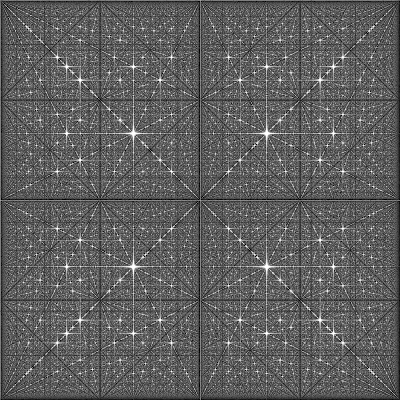### Fourier transform of the coprime numbers map

Disclaimer: the idea is not mine, I've found a low-resolution image and the idea behind it here.

### Co-prime integers

A pair of integers is called co-prime, if their greatest common denominator is 1. For example, 4 and 21 are co-prime, while 14 and 21 are not.

First let's draw a map of co-prime pairs. The image below shows co-prime pairs with white color.Map of the co-prime integers, (0,0) is at the top-left corner.

The map above clearly shows some regularities, but it is actually aperiodic. It is known that its average density is $$6 / \pi^2$$, or about 61%.

### Fourier transform

But what if you take a Fourier transform of this nearly periodic pattern? The result is the following mind-blowing image.Logarithmic amplitude of 2d Fourier transform of the co-prime numbers map, 512x512 image.

Fourier transform discovers periodicity in the source data, so applying it to some data points with nearly periodic patterns can often produce interesting results. For example, see Fourier transform of the Hilbert curve images. Still, the 3-dimensional look of the resulting image is something totally unexpected for me. Below is the same image, but rendered for a bigger fragment of the plane, 2048x2048.Logarithmic amplitude of the 2d Fourier transform of the co-prime numbers map, 2048x2048 image.

Finally, for those who like it big, here is a 4096x4096 image, 15 Mb large. Open the link and click "Download" to get the whole file.

### Extensions

The idea can be extended by building the co-prime matrix not for integers but for some general integer sequence. This gallery contains some of the spectra, obtained for different sequences: Fibonacci numbers, $$n^2+1$$, $$n^3+3$$, $$n^2-n+41$$ (Euler polynomial with many primes), partition function values and tribonacci numbers. Curious fact: the spectrum for the Fibonacci numbers is very similar visually to the original integers spectrum. But there is a difference, the image below shows two spectra superimposed, with yellow color for integers, and blue color for Fibonacci numbers.

### Source code

The images above were generated by a simple Python script below. See it here, if the gist fails to load. To run it, you will need to install Numpy and PIL (Python Imaging Library). The code itself is fairly straightforward, in fact, the most complicated part is the adaptive calculation of the brightness diapason.Matilde said…
Hello, I would like to ask permission to reproduce your image "Logarithmic amplitude of 2d Fourier transform of the co-prime numbers map" in a book I am writing. What do I need to do? You can find me here: http://www.its.caltech.edu/~matilde/@Matilde, sorry, blogger did not send me notification. All my images are CC0, so you can do whatever you like with them. It would be cool if you mention me somehow, but you don't have to - just take it and use it. In any case, this image is not my discovery.Matilde said…
Dear Dmitry,
Thanks a lot! Of course I will mention you as the author of the image.
Best wishes, Matilde